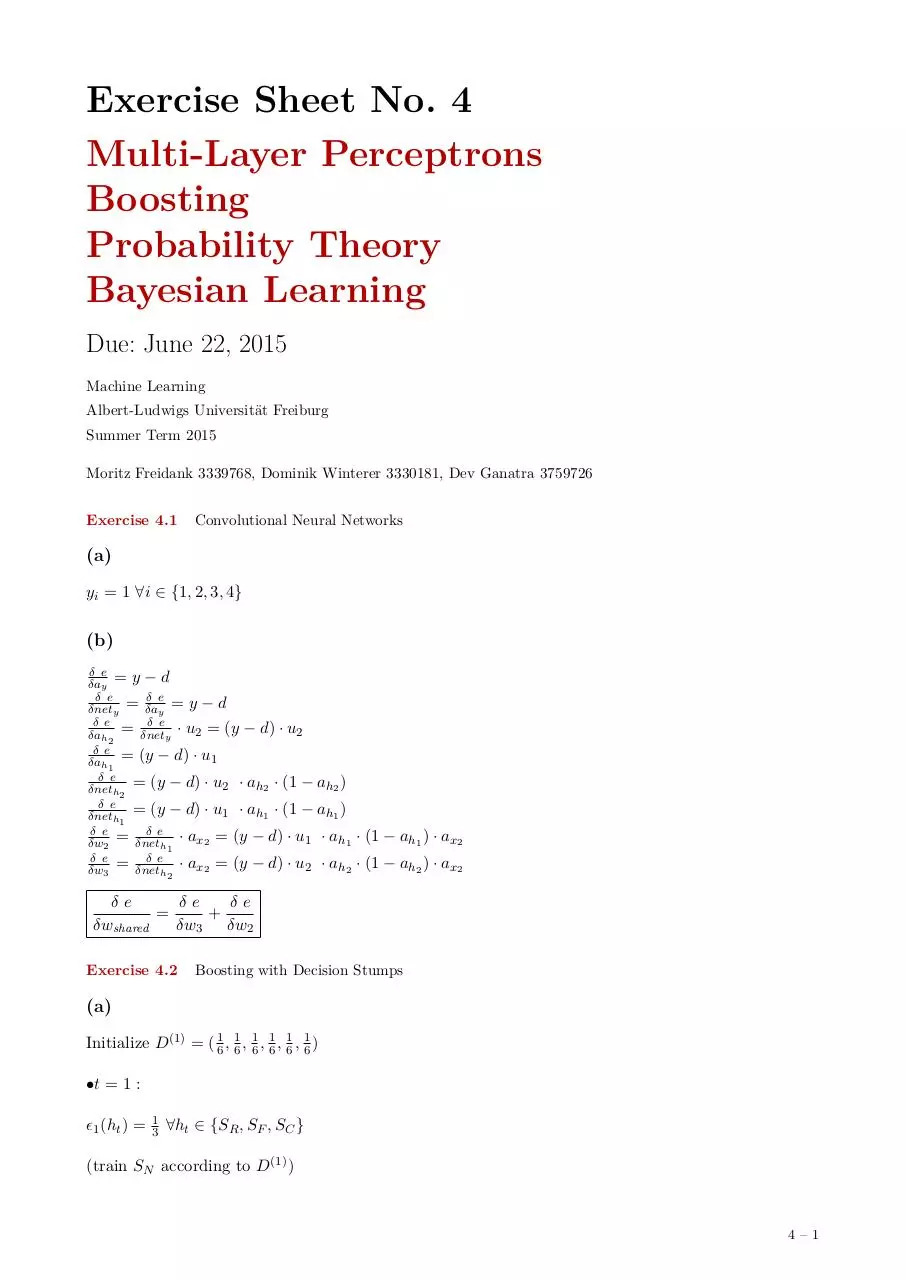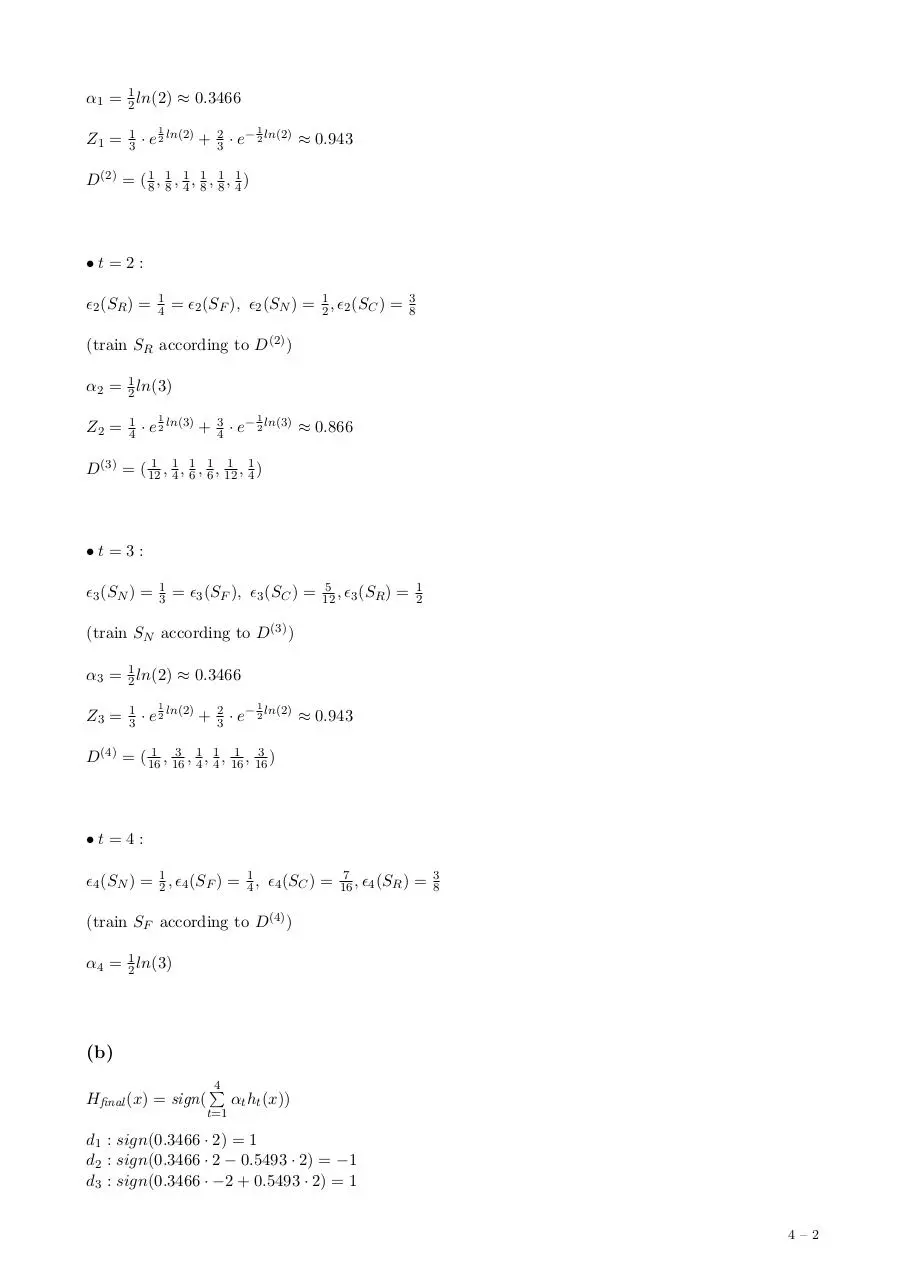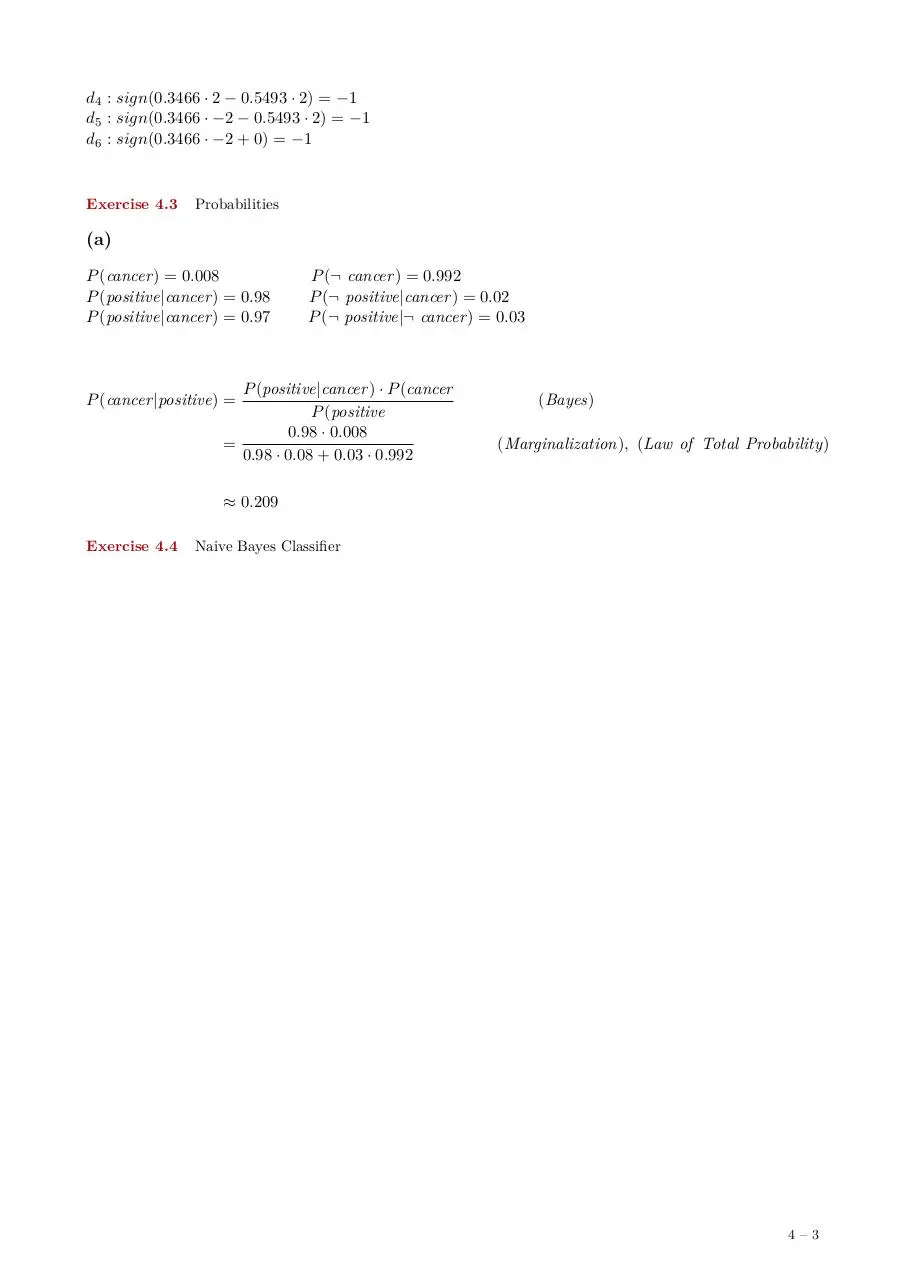# ML EX04 MFDWDG .pdf

### File information

This PDF 1.5 document has been generated by LaTeX with hyperref package / pdfTeX-1.40.14, and has been sent on pdf-archive.com on 25/08/2015 at 22:42, from IP address 84.169.x.x. The current document download page has been viewed 411 times.
File size: 227.8 KB (3 pages).
Privacy: public file

### Document preview

Exercise Sheet No. 4
Multi-Layer Perceptrons
Boosting
Probability Theory
Bayesian Learning
Due: June 22, 2015
Machine Learning
Albert-Ludwigs Universität Freiburg
Summer Term 2015
Moritz Freidank 3339768, Dominik Winterer 3330181, Dev Ganatra 3759726
Exercise 4.1

Convolutional Neural Networks

(a)
yi = 1 ∀i ∈ {1, 2, 3, 4}

(b)
δ e
δay = y − d
δ e
δ e
δnety = δay = y − d
δ e
δ e
δah2 = δnety · u2 = (y − d) · u2
δ e
δah1 = (y − d) · u1
δ e
δneth2 = (y − d) · u2 · ah2 · (1 −
δ e
δneth1 = (y − d) · u1 · ah1 · (1 −
δ e
δ e
δw2 = δneth1 · ax2 = (y − d) · u1
δ e
δ e
δw3 = δneth · ax2 = (y − d) · u2

ah2 )
ah1 )

2

· ah1 · (1 − ah1 ) · ax2
· ah2 · (1 − ah2 ) · ax2

δe
δe
δe
=
+
δwshared
δw3 δw2
Exercise 4.2

Boosting with Decision Stumps

(a)
Initialize D(1) = ( 16 , 61 , 61 , 61 , 16 , 16 )
•t = 1 :
1 (ht ) =

1
3

∀ht ∈ {SR , SF , SC }

(train SN according to D(1) )

4–1

α1 = 21 ln(2) ≈ 0.3466
Z1 =

1
3

1

· e 2 ln(2) +

2
3

1

· e− 2 ln(2) ≈ 0.943

D(2) = ( 18 , 18 , 41 , 18 , 81 , 14 )

•t=2:
2 (SR ) =

1
4

= 2 (SF ), 2 (SN ) = 12 , 2 (SC ) =

3
8

(train SR according to D(2) )
α2 = 21 ln(3)
Z2 =

1
4

1

· e 2 ln(3) +

3
4

1

· e− 2 ln(3) ≈ 0.866

1 1 1 1 1 1
D(3) = ( 12
, 4 , 6 , 6 , 12 , 4 )

•t=3:
3 (SN ) =

1
3

= 3 (SF ), 3 (SC ) =

5
12 , 3 (SR )

=

1
2

(train SN according to D(3) )
α3 = 21 ln(2) ≈ 0.3466
Z3 =

1
3

1

· e 2 ln(2) +

2
3

1

· e− 2 ln(2) ≈ 0.943

1 3 1 1 1 3
, 16 , 4 , 4 , 16 , 16 )
D(4) = ( 16

•t=4:
4 (SN ) = 21 , 4 (SF ) = 14 , 4 (SC ) =

7
16 , 4 (SR )

=

3
8

(train SF according to D(4) )
α4 = 12 ln(3)

(b)
Hfinal (x) = sign(

4
P

αt ht (x))

t=1

d1 : sign(0.3466 · 2) = 1
d2 : sign(0.3466 · 2 − 0.5493 · 2) = −1
d3 : sign(0.3466 · −2 + 0.5493 · 2) = 1
4–2

d4 : sign(0.3466 · 2 − 0.5493 · 2) = −1
d5 : sign(0.3466 · −2 − 0.5493 · 2) = −1
d6 : sign(0.3466 · −2 + 0) = −1

Exercise 4.3

Probabilities

(a)
P (cancer) = 0.008
P (positive|cancer) = 0.98
P (positive|cancer) = 0.97

P (¬ cancer) = 0.992
P (¬ positive|cancer) = 0.02
P (¬ positive|¬ cancer) = 0.03

P (positive|cancer) · P (cancer
P (positive
0.98 · 0.008
=
0.98 · 0.08 + 0.03 · 0.992

P (cancer|positive) =

(Bayes)
(Marginalization), (Law of Total Probability)

≈ 0.209
Exercise 4.4

Naive Bayes Classifier

4–3ML_EX04_MFDWDG.pdf (PDF, 227.8 KB)

### Share on social networks

#### HTML Code

Copy the following HTML code to share your document on a Website or Blog